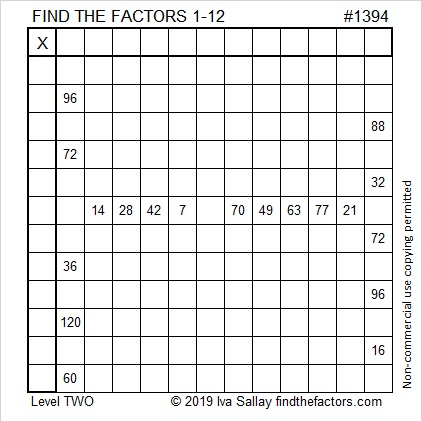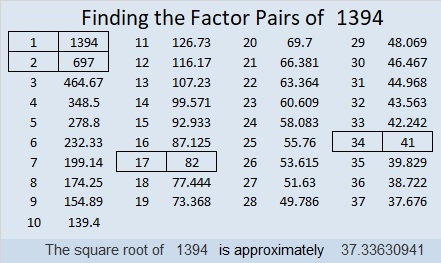# 1394 and Level 2

The factors and most of the products are missing from this multiplication table, and the ones that are there aren’t in there usual places. Can you figure out where everything goes?Print the puzzles or type the solution in this excel file: 12 Factors 1389-1403

Now I’ll write a little bit about the puzzle number, 1394:

• 1394 is a composite number.
• Prime factorization: 1394 = 2 × 17 × 41
• 1394 has no exponents greater than 1 in its prime factorization, so √1394 cannot be simplified.
• The exponents in the prime factorization are 1, 1, and 1. Adding one to each exponent and multiplying we get (1 + 1)(1 + 1)(1 + 1) = 2 × 2 × 2 = 8. Therefore 1394 has exactly 8 factors.
• The factors of 1394 are outlined with their factor pair partners in the graphic below.1394 is the hypotenuse of FOUR Pythagorean triples:
306-1360-1394 which is (9-40-41) times 34
370-1344-1394 which is 2 times (185-672-697)
656-1230-1394 which is (8-15-17) times 82
910-1056-1394 which is 2 times (455-528-697)